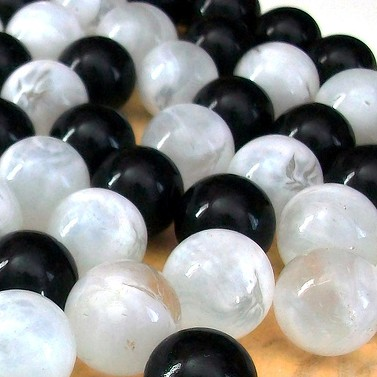A Gee-Whiz Probability Problem

Probability Level 3There are $10$ identical balls of each colour, black and white, evenly mixed in the urn. If I randomly take $3$ balls at once without looking, what is the probability of getting at least two balls of the same color in percent $(\%)$?

×